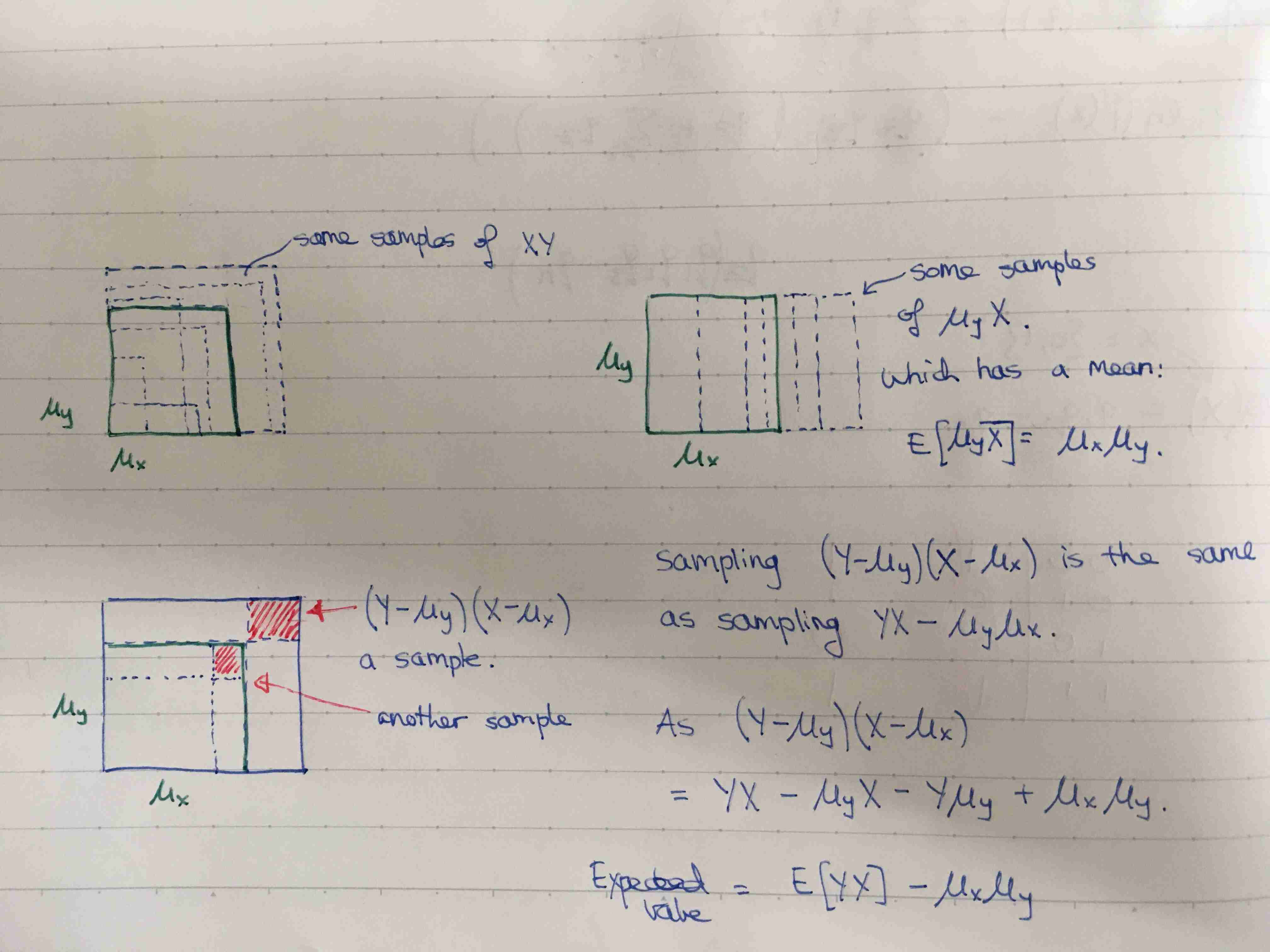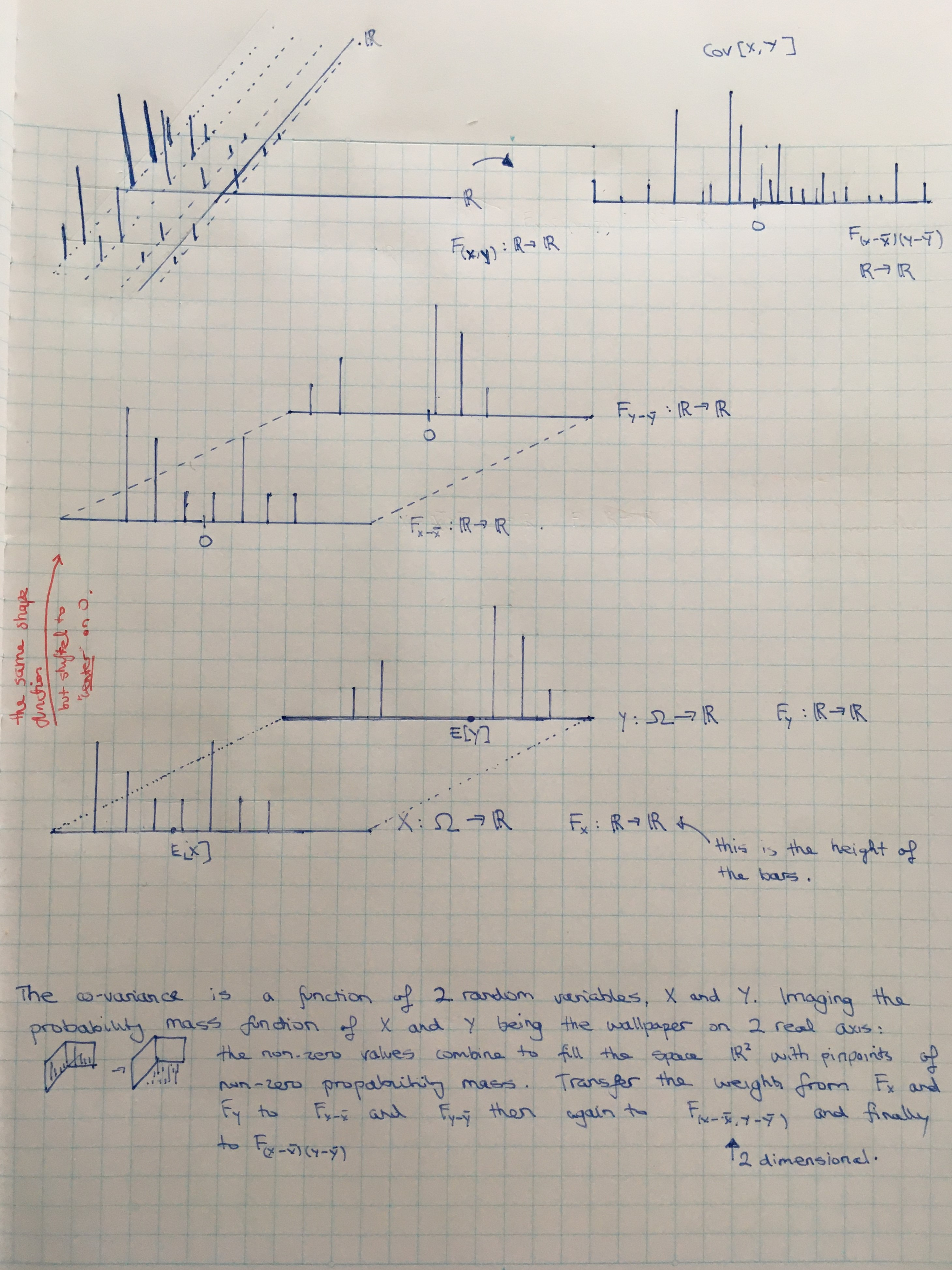Show Question
Math and science::INF ML AI

# Covariance

Let $$(\Omega, \mathrm{F}, \mathbb{P})$$ be a discrete probability space let $$X: \Omega \to S_x$$ and $$Y : \Omega \to S_y$$, be two random variables, where $$S_x$$ and $$S_y$$ are finite subsets of $$\mathbb{R}$$. Then the covariance of $$X$$ and $$Y$$ is defined as the mean of the following random variable:

$Z = (X - \mathrm{E}[X])(Y - \mathrm{E}[X])$

In terms of $$X$$ and $$Y$$, the calculation for covariance is thus:

$\mathrm{Cov}[X, Y] = \sum_{\omega \in \Omega} p(\omega) (X(\omega) - \mathrm{E}[X])(Y(\omega) - \mathrm{E}[Y]))$

Covariance is a real that gives some sense of how much two variables are linearly related to each other, as well as the scale of these variables. Covariance is a function of a probability space and two random variables.

In it's most reduced form, a random variable that is the product of two other random variables is what fully determines covariance, which can be seen as the mean of this variable. $$X \cdot Y : \Omega \to S_x \times S_y$$. Then, covariance is a can be considered a function of this random variable. The expectation can be carried out by summing over the product space, and using the probability $$P(x, y)$$ which can be considered syntax for $$\mathbb{P}((X \times Y)^{-1}((x, y))$$, where $$\mathbb{P}$$ is the function in $$(\Omega, \mathrm{F}, \mathbb{P} )$$:

\begin{align*} \operatorname{Cov}(X, Y) &= \mathrm{E}[(X - \mathrm{E}[X])(Y - \mathrm{E}[Y])] \\ \operatorname{Cov}(X, Y) &= \sum_{x \in S_x, y \in S_y} P(x, y)(x - \mathrm{E}[X])(y - \mathrm{E}[Y]) \\ \end{align*}

### Not just two probability distributions

It's important to note how you can't tell if two random variables are independent just by looking at both of their probability distributions. What is important is if there is some relationship between where $$\omega$$ is sent by the function $$X$$ and where it is sent by $$Y$$.

### Interpreting the number

High absolute values of the covariance mean that the values change very much and are both far from their respective means at the same time. If the sign of the covariance is positive, then both variables tend to take on high values simultaneously. If the sign is negative, then one variable tends to taken on a relatively high value at the times where the other takes on a relatively low value and visa versa.

#### Independence and covariance

Two random variables that are independence have zero covariance! :o

This is a “implies” statement, and not a iff statement. (Dependent variables can have zero covariance).

#### Relation to correlation

The correlation, another measure, normalized the contribution of each variable in order to remove the effect of scale.

#### Alternative form

Covariance has another form:

$\operatorname{Cov}(X, Y) =\mathrm{E}[XY] - \mathrm{E}[X]\mathrm{E}[Y]$

Some visual reasoning:Covariance (and many operations on distributions) can be best understood by rooting one's focus on the distribution of a 1D random variable just before an operation such as taking an expectiation. For covariance, this means focusing on the random variable $$(X - \overline{X})(Y - \overline{Y})$$ and it's distribution,  $$F_{(X - \overline{X})(Y - \overline{Y})}$$, which is a function $$\mathbb{R} \to \mathbb{R}$$; covariance is the mean of this random variable. Start stepping backwards by seeing each value of $$F_{(X - \overline{X})(Y - \overline{Y})}$$ as having collected it's weights from the 2D distribution $$F_{(X - \overline{X}, Y - \overline{Y})}$$...notice the comma there! These weights are the result of a sort of cartesian product of the distributions $$F_{X - \overline{X}}$$ and $$F_{Y - \overline{Y}}$$.

There seems to be a typo in the below image. $$F_{(X,Y)}$$ should have domain $$\mathbb{R} \times \mathbb{R}$$, not $$\mathbb{R}$$.### More than 3 variables

The covariance of a random vector $$x \varepsilon R^n$$ is an n x n matrix, such that $$Cov(x)_{i,j} = Cov(x_i, x_j)$$. The diagonal elements of the covariance matrix gives the variances of each vector element.